# Adding Problems

#### Number of problems found: 362

• Adding mixed 42 and 1 8th plus 1 and 1 3rd =
• Adding mixed numerals3 3/4 + 2 3/5 + 5 1/2 Show your solution.
• Adding shapes5 triangles + 1 square = how many sides in all
• Adding mixed 3Why does 1 3/4 + 2 9/10 equal 4.65? How do you solve this?
• Time addingAdd and write the result again as hours, minutes, seconds: 2hodiny45min15s + 1h20m50s =
• Adding mixed numbersAdd this two mixed numbers: 1 5/6 + 2 2/11=
• Temperature addingAt a weather centre, the temperature at midnight was -2 degree Celsius and by noon it had raised 4 degree Celsius. What is the new temperature?
• Hello adding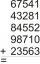Fill letters instead of digits so the indicated sum (equal letters represent equal digits). What number is hidden under the letter J? A A H A H O A H O J -------------------------- 4 3 2 1
• Expression 1What is 7+8-(5×2)+5-4+(6×(5-3)+6)-(8+10)-7+6?
• Integer add to fraction7 is added to the sum of 4/5 and 6/7
• Evaluate expressionIf x=2, y=-5 and z=3 what is the value of x-2y
• Combine / add termCombine like terms 4c+c-7c
• How many 3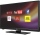How many hours the Andersons watched TV in all Wednesday 3/1 hr Thursdays 2/3 hr Friday 4/5 hr Saturday 3/4 hr
• WhichWhich decimals when subtracted equal 3.89: a - b = 3.89
• Brackets 2Add parenthesis to make true: 5-2×6-4+2=5
• Televisions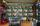Television is the fifth most expensive and tenth cheapest TV. How many different TVs are in stores?
• Operations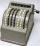Sum of the numbers 1.01 and 3.35 multiply by the difference of numbers 6.69 and 1.39.
• Average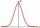The average of 5 numbers is 108. What is its sum?
• Multiples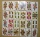What is the sum of the multiples of number 7 that are greater than 30 but less than 56?
• Two numbersFind the four times smaller number for numbers 12 and 36 and then add them.

Do you have an interesting mathematical word problem that you can't solve it? Submit a math problem, and we can try to solve it.

We will send a solution to your e-mail address. Solved examples are also published here. Please enter the e-mail correctly and check whether you don't have a full mailbox.

Please do not submit problems from current active competitions such as Mathematical Olympiad, correspondence seminars etc...